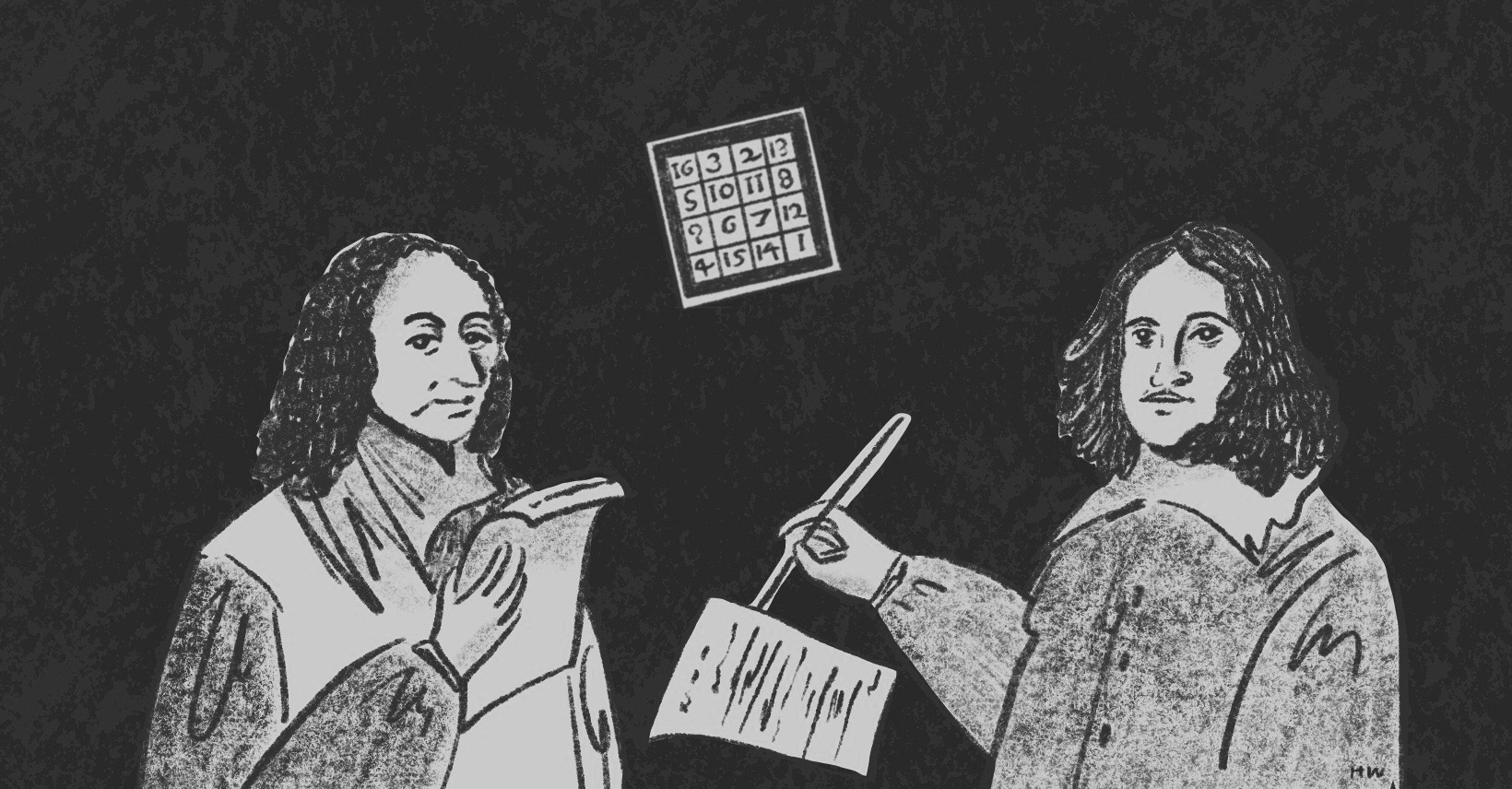×資料管理 統計分析 相關資源 巨人肩膀 語法索引 關於作者

## 簡介

• rake() -多變數反覆加權
• anesrake() -多變數反覆加權
• svydesign() -宣告複雜抽樣調查資料
• svytable() -複雜抽樣資料交叉表
• svygofchisq() -複雜抽樣資料卡方適合度檢定
• stack() -堆疊交叉表
• postStratify() -事後分層加權
• calibrate() -GREG一般回歸校正

19歲以下 20-39歲 40-59歲 60歲以上 邊際%

## 多變數反覆加權

Deming與Stephan(1940)第一次提出反覆加權的概念後，它有不同的名稱包含sample-balancing、raking ratio estimation、iterative proportional fitting，不過最常用的名稱還是Raking。

$W_{ij}=\frac{N_{ij}}{N} \times \frac{n}{n_{ij}}$

$$W_{female}=\frac{50}{100} \times \frac{100}{60}=0.83$$

$$W_{male}=\frac{50}{100} \times \frac{100}{40}=1.25$$

R有兩個重要的延伸套件survey、anesrake可以處理加權，尤其是University of Auckland生物統計學教授Thomas Lumley開發的survey更是處理加權資料分析的重要套件，其介紹可參考Lumley成立的Survey analysis in R。我們現在就以虛擬資料gender_age.csv來示範如何用survey與anesrake進行多變數反覆加權。

 > example<-read.csv("c:/Users/USER/Downloads/gender_age.csv", header=T, sep=",") > head(example) gender age 1 1 1 2 1 1 3 1 1 4 1 1 5 1 2 6 1 2 

 > example$gender<-factor(example$gender, levels=c(1,2), labels=c("Male","Female")) > example$age<-factor(example$age, levels=c(1,2,3,4), labels=c("Under 19","20-39","40-59","Over 60")) > table(example$gender) Male Female 39 61 > table(example$age) Under 19 20-39 40-59 Over 60 11 41 36 12 

 > chisq.test(table(example$gender), p=c(0.495, 0.505)) Chi-squared test for given probabilities data: table(example$gender) X-squared = 4.4104, df = 1, p-value = 0.03572 > chisq.test(table(example$age), p=c(0.171, 0.273, 0.316, 0.240)) Chi-squared test for given probabilities data: table(example$age) X-squared = 15.664, df = 3, p-value = 0.001329 

### survey套件

survey其實是用來處理複雜抽樣設計(complex survey design)的套件。我們常見熟悉的統計方法是建立在簡單隨機抽樣每個樣本有相同機率分布、相同權重的假設上，但實務上這種獨立同分布(independent and identically distributed, i.i.d)的假設碰到分層抽樣、集群抽樣、加權等作法已經違反，這種複雜抽樣所獲得的樣本變異數通常會較簡單隨機抽樣來得大，所以用一般統計方法分析會導致估計錯誤，才會衍生出複雜抽樣調查的資料分析。survey就是R專門處理複雜抽樣調查的分析套件。

 > library(survey) > unweighted<-svydesign(ids=~0, data=example) > gender_p<-data.frame(gender=c("Male","Female"), Freq=nrow(example)*c(0.495,0.505)) > age_p<-data.frame(age=c("Under 19","20-39","40-59","Over 60"), Freq=nrow(example)*c(0.171,0.273,0.316,0.240)) 

 > raking<-rake(design=unweighted, sample.margins=list(~gender, ~age), population.margins=list(gender_p, age_p)) > stack(table(weights(raking))) values ind 1 23 0.483958072437301 2 21 0.646990276173929 3 18 0.898275796330115 4 7 1.18549042351196 5 15 1.20088028002317 6 10 1.75026554378407 7 4 2.20039175885406 8 2 3.24867228107965 

 > example$weights<-weights(raking) > example gender age weights 1 Male Under 19 2.2003918 2 Male Under 19 2.2003918 3 Male Under 19 2.2003918 4 Male Under 19 2.2003918 5 Male 20-39 0.8982758 6 Male 20-39 0.8982758 ⋮ 98 Female Over 60 1.7502655 98 Female Over 60 1.7502655 100 Female Over 60 1.7502655  有了每個個案的權重後，我們再一次用svydesign()將example宣告為加權後的樣本，以利比較加權前與加權後的樣本分配。原本樣本結構中男性偏少的問題經加權調整後已獲得解決，男性與女性的比例接近50%：50%。  > svytable(~gender+age, unweighted) #加權前 age gender Under 19 20-39 40-59 Over 60 Male 4 18 15 2 Female 7 23 21 10 > weighted<-svydesign(id=~0, data=example, weights=~weights) > summary(svytable(~gender+age, design=weighted)) #加權後 age gender Under 19 20-39 40-59 Over 60 Male 9 16 18 6 Female 8 11 14 18  以survey套件裡的svygofchisq()進行卡方適合度檢定，p值>.05顯示資料經過加權處理後與母體結構一致。  > svygofchisq(~gender, p=c(0.495,0.505), design=weighted) Design-based chi-squared test for given probabilities data: ~gender X-squared = 1.432e-05, scale = 1.3262, df = 1.0000, p-value = 0.9974 > svygofchisq(~age, p=c(0.171, 0.273, 0.316, 0.240), design=weighted) Design-based chi-squared test for given probabilities data: ~age X-squared = 0, scale = 1.9046, df = 2.3967, p-value = 1  ### anesrake套件 anesrake是設計用來加權美國全國大選(American National Election Studies)的套件，雖然不像survey有分析複雜抽樣設計的功能，但一樣可以對資料進行加權，指令就是anesrake()。用anesrake()對資料加權前，必須先將母體分布轉換為表單形式。  > library(anesrake) > gender_target<-c(0.495, 0.505) > age_target<-c(0.171, 0.273, 0.316, 0.240) > names(gender_target)<-c("Male","Female") > names(age_target)<-c("Under 19", "20-39", "40-59", "Over 60") > target<-list(gender_target, age_target) > names(target)<-c("gender", "age") > target$gender Male Female 0.495 0.505 $age Under 19 20-39 40-59 Over 60 0.171 0.273 0.316 0.240  接下來因為anesrake()指令的需要，必須賦予每個樣本一個編號。所以我們創造出一個名為id的變數，從1開始編碼到100，然後與原本的資料作結合。  > id<-1:length(example$age) > example<-cbind(example, id) > head(example) gender age weights id 1 Male Under 19 2.2003918 1 2 Male Under 19 2.2003918 2 3 Male Under 19 2.2003918 3 4 Male Under 19 2.2003918 4 5 Male 20-39 0.8982758 5 6 Male 20-39 0.8982758 6 

 > raking2<-anesrake(target, example, caseid=example$id, choosemethod="total")  "Raking converged in 16 iterations"  用weightvec參數並配合stack()指令整理經過16次迭代後的權值如下：  > stack(table(raking2$weightvec)) values ind 1 23 0.48372580975453 2 21 0.646691420427651 3 18 0.898572576424768 4 7 1.18499595188209 5 15 1.20129867806796 6 10 1.74988148834905 7 4 2.20125708420635 8 2 3.25059255825473 

 > example$weights2<-raking2$weightvec 

## 事後分層加權

19歲以下 20-39歲 40-59歲 60歲以上

 > genderXage<-table(example$gender, example$age) > addmargins(prop.table(genderXage)) Under 19 20-39 40-59 Over 60 Sum Male 0.04 0.18 0.15 0.02 0.39 Female 0.07 0.23 0.21 0.10 0.61 Sum 0.11 0.41 0.36 0.12 1.00 

 > strata<-c("") > strata[example$gender=="Male" & example$age=="Under 19"]<-1 > strata[example$gender=="Male" & example$age=="20-39"]<-2 > strata[example$gender=="Male" & example$age=="40-59"]<-3 > strata[example$gender=="Male" & example$age=="Over 60"]<-4 > strata[example$gender=="Female" & example$age=="Under 19"]<-5 > strata[example$gender=="Female" & example$age=="20-39"]<-6 > strata[example$gender=="Female" & example$age=="40-59"]<-7 > strata[example$gender=="Female" & example$age=="Over 60"]<-8 > strata<-factor(strata, levels=c(1,2,3,4,5,6,7,8), > example<-cbind(example, strata) 

 > joint_population<-data.frame(strata=c(1,2,3,4,5,6,7,8), + percent=c(0.089, 0.140, 0.155, 0.111, 0.082, 0.133, 0.161, 0.129)) > joint_population strata percent 1 1 0.089 2 2 0.140 3 3 0.155 4 4 0.111 5 5 0.082 6 6 0.133 7 7 0.161 8 8 0.129 

 > unweighted2<-svydesign(ids=~0, data=example) > ps<-postStratify(design=unweighted2, strata=~strata, population=joint_population) 

 > stack(table(weights(ps))) values ind 1 23 0.00578260869565217 2 21 0.00766666666666667 3 18 0.00777777777777778 4 15 0.0103333333333333 5 7 0.0117142857142857 6 10 0.0129 7 4 0.02225 8 2 0.0555 > example\$weights3<-weights(ps) 

 > psweighted<-svydesign(id=~0, data=example, weights=~weights3) > svytable(~gender+age, design=psweighted) age gender Under 19 20-39 40-59 Over 60 Male 0.089 0.140 0.155 0.111 Female 0.082 0.133 0.161 0.129 

 > pop_percent<-c((Intercept)=0.089, "2"=0.140, "3"=0.155, "4"=0.111, "5"=0.082, "6"=0.133, "7"=0.161, "8"=0.129) > GREG<-calibrate(unweighted2, ~strata, pop_percent) > stack(table(weights(GREG))) values ind 1 4 -0.2055 2 23 0.00578260869565228 3 21 0.00766666666666693 4 18 0.00777777777777788 5 15 0.0103333333333333 6 7 0.0117142857142859 7 10 0.0129000000000001 8 2 0.0555000000000001 

 > GREG2<-calibrate(unweighted2, ~strata-1, pop_percent) > stack(table(weights(GREG2))) values ind 2 23 0.00578260869565217 3 21 0.00766666666666671 4 18 0.00777777777777777 5 15 0.0103333333333333 6 7 0.0117142857142857 7 10 0.0128999999999999 1 4 0.02055 8 2 0.0555